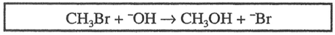Problem: Consider this prototypical nuclephilic substitution shown in the box. The effect of doubling the volume of solvent would be to multiply the reaction rate by a factor of  a) 1/4 b) 1/2 c) 2 d) 4

FREE Expert Solution
90% (406 ratings)
Problem Details

Consider this prototypical nuclephilic substitution shown in the box. The effect of doubling the volume of solvent would be to multiply the reaction rate by a factor of

a) 1/4

b) 1/2

c) 2

d) 4What scientific concept do you need to know in order to solve this problem?

Our tutors have indicated that to solve this problem you will need to apply the Rate Calculations concept. If you need more Rate Calculations practice, you can also practice Rate Calculations practice problems .

What is the difficulty of this problem?

Our tutors rated the difficulty of Consider this prototypical nuclephilic substitution shown in... as medium difficulty.

How long does this problem take to solve?

Our expert Organic tutor, Jonathan took 2 minutes to solve this problem. You can follow their steps in the video explanation above.

What professor is this problem relevant for?

Based on our data, we think this problem is relevant for Professor Jose's class at UHD.